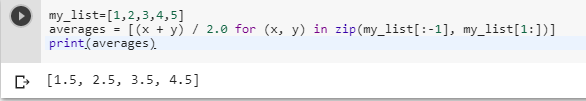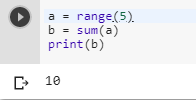2 views
in Python

I have a list of numbers such as [1,2,3,4,5...], and I want to calculate (1+2)/2 and for the second, (2+3)/2 and the third, (3+4)/2, and so on. How can I do that?

I would like to sum the first number with the second and divide it by 2, then sum the second with the third and divide by 2, and so on.

Also, how can I sum a list of numbers?

a = [1, 2, 3, 4, 5, ...]

Is it:

b = sum(a)

print b

to get one number?

This doesn't work for me.

by (106k points)
edited by

As you want to add  (element 0 + element 1) / 2, (element 1 + element 2) / 2, ... etc:-

To do the sum of elements we will make two lists: The first list will contain one of every element except the first, and the second list will contain one of every element except the last. Then for calculating the averages, we will use zip to take pairs from two lists.

my_list=[1,2,3,4,5]

averages = [(x + y) / 2.0 for (x, y) in zip(my_list[:-1], my_list[1:])]

print(averages)In your second question you want to sum all the list values so you can do that by using the following piece of code:

a = range(10)

b = sum(a)

print(b)